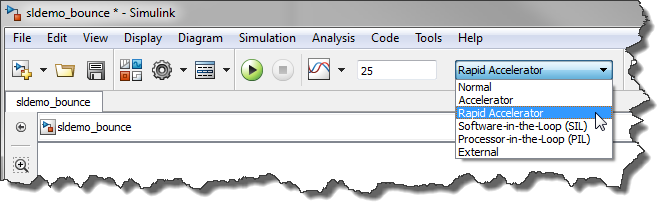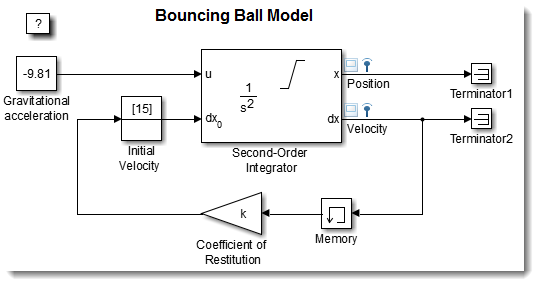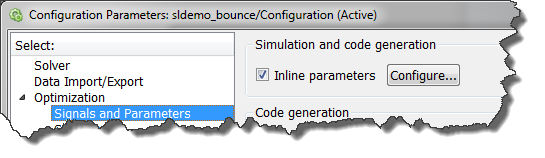# Getting the most out of Rapid Accelerator mode

When working with Simulink, it's important to configure your model and workflows to be as efficient as possible for the task you are working on.

Today I will show how to setup a model to get the maximum performance when you need to run many simulations and vary parameters. This is often the case if you do monte-carlo simulation, or system optimization.

Rapid Accelerator

As explained here, the rapid accelerator mode can speed up simulation by generating an executable for your model. The speedup can vary between models for various reasons. Personally, I have seen some models run more than 10 times faster in rapid accelerator mode.RapidAcceleratorUpToDateCheck off

When you click the play button in a model or use the sim command, Simulink verifies if your model has changed or not. If the model has not changed structurally, the rapid accelerator executable is not re-generated.

One nice thing with rapid accelerator is that, once the executable has been generated, it is possible to skip this part where Simulink verifies if the model has changed or not. For large models, this can save a lot of time, bringing the initialization time to zero.

Let's see how this can be done using a simple demo like sldemo_bounce where I want to vary the coefficient of restitution, which I specified as k in the block dialog.If I want to be able to tune the value of k, I need to define it as a Simulink.Parameter object:

k = Simulink.Parameter
k.Value = -0.9;


and I enable the Inline Parameters option in the model configuration.Once this is done, I explicitly build the rapid accelerator executable:

mdl = 'sldemo_bounce';


As you can see, this returns a structure containing all the information relevant to tunable parameters in the model. Using this structure, we can create an array of run-time parameter structures for all the values we want to run:

k_values = [-0.9:0.1:-0.1];
for i = 1:length(k_values)
paramSet(i) = Simulink.BlockDiagram.modifyTunableParameters(rtp, 'k', k_values(i));
end


And we are ready to simulate, by passing to the sim command, we pass the parameters RapidAcceleratorUpToDateCheck and RapidAcceleratorParameterSets.

for i = 1:length(k_values)
simout(i) = sim(mdl,'SimulationMode','rapid',...
'RapidAcceleratorUpToDateCheck','off', ...
'RapidAcceleratorParameterSets',paramSet(i));
end


Update: In MATLAB R2017a the function PARSIM got introduced. For a better experience simulating models in a loop (in series or parallel), we recommend using PARSIM instead of SIM inside for/parfor. See the more recent blog post Simulating models in parallel made easy with parsim for more details.

Now it's your turn

Give that a try and let us know what you think by leaving a comment here.

|

### 댓글

댓글을 남기려면 링크 를 클릭하여 MathWorks 계정에 로그인하거나 계정을 새로 만드십시오.